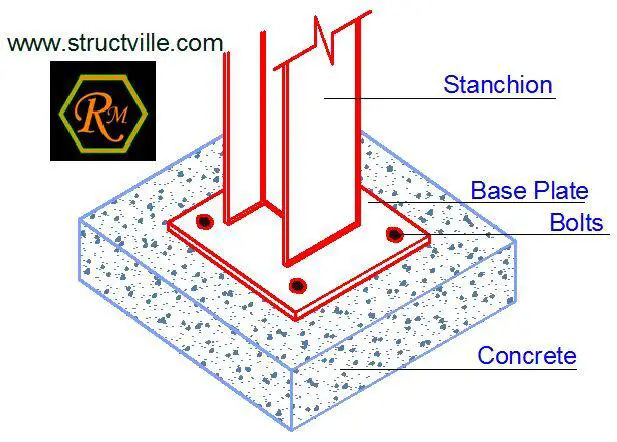# Design of Column Base Plate Connections (EC3)This article is aimed at providing the procedure for the design of column base plates subjected to axial and shear forces according to Eurocode  3. Find a design example below.

Problem Statement
It is required to specify the appropriate thickness of a base plate to support a UC 203 x 203 x 60 subjected to the following loads. The connection is assumed to be pinned with four bolts outside the column profile.
The supporting concrete is to have a grade fck = 25 N/mm2

Characteristic force from permanent action Gk = 620 kN
Characteristic force from leading variable action  Qk = 132 kN

Partial Factor for loads (BS EN 1990 NA 2.2.3.2 Table NA.A1.2(B))
Permanent action γG = 1.35 (unfavourable)
Variable action γG = 1.5
Reduction factor ξ = 0.925

At ultimate limit state;
NEd = 1.35Gk + 1.5Qk
NEd = 1.35(620) + 1.5(132) = 1035 kN

Column Dimensions and Properties
Depth (d) = 209.6mm
Width (b) = 205.8mm
Thickness of web (tw) = 9.4mm
Thickness of flange = (tf) = 14.2mm
Perimeter of section = 1206.4mm
Area of section = 76.4 cm2

Partial factor for resistance
γm0 = 1.0
γm1 = 1.25

Base Plate Details
Strength of concrete = 25 N/mm2
Foundation bearing strength fcd = αccfckc
Take αcc = 0.85 (for axial compression)
γc = 1.5

Therefore;
fcd = (0.85 × 25)/1.5 = 14.167 N/mm2

Area of base plate required Ac0 = NEd / fcd = (1035 × 1000)/14.167 = 73057.104 mm2

Effective Area = 4c2 + (Section Perimeter × c) + Section Area

Where c is the cantilever outstand of the effective area. This is shown below;

73057.104  = 4c2 + 1206.4c + 7640
On solving;

c = 46.924 mm

Check;
(h – 2tf)/2 = (209.6 – 2 × 14.2) / 2 = 90.6mm
Since 90.6mm > 46.924 mm, there is no overlap between the flanges.

Thickness of base plate (tp)
tp = c[3fcd / (fyγm0)]0.5

tp = 46.924 × [(3 × 14.167) / (275 × 1.0)]0.5 = 18.447 mm

Therefore provide a base plate of thickness tp = 20 mm in S275 material (since tp is less than 40mm).

Connection of base plate to column
It is assumed that the axial force is transferred by direct bearing, which is achieved by normal fabrication processes. Only nominal welds are required to connect the base plate to the column, though in practice full profile 6mm fillet welds are often used.

Thank you visiting Structville today!!!